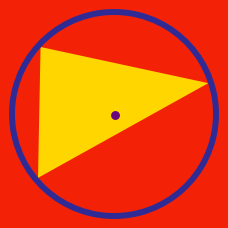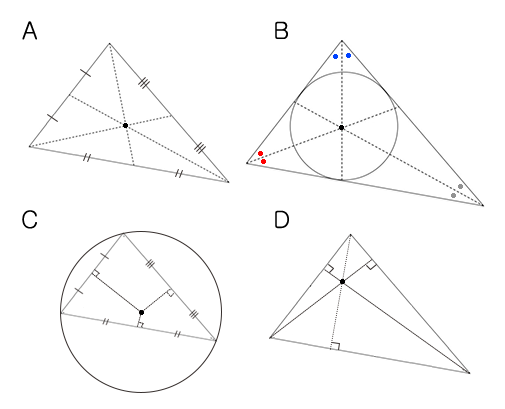Geometry

# Triangles - Circumcenter

The circumcenter is the intersection of which 3 lines in a triangle?

Which of the following triangles has its circumcenter outside of it?Which diagram shows a circumcenter?

Given the coordinates of the vertices of a triangle, how can we get the circumcenter of the triangle?

In triangle $ABC$, $A = (0,0)$, $B = (0, 4 )$ and $C = (14, 0)$. What are the coordinates of $O$, the circumcenter of triangle $ABC$?

×